### Home > CALC > Chapter 3 > Lesson 3.2.3 > Problem3-78

3-78.
1. The position of a ball as a function of time is given by the function below where s(t) is in meters and t is in seconds. Homework Help ✎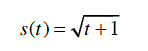1. Use your calculator to approximate the instantaneous velocity of the ball at 1, 5, 10, and 100 seconds.

2. What do you predict happens to the velocity of the ball after a very long time (i.e. as t→∞)?

3. What happens to the position of the ball after a very long time, (i.e. what is s(t))? Does this make sense given your answer to part (b)?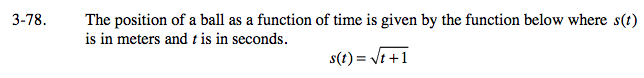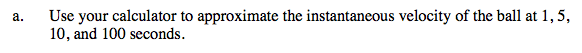Instantaneous velocity = Instantaneous Rate of Change (IROC) = Derivative

$\text{Rewrite }s(t)\text{ using exponents: }s(t)=(t+1)^{1/2}$

Find s'(t) using the Power rule.

Evaluate s'(1), s'(5), s'(10) and s'(100).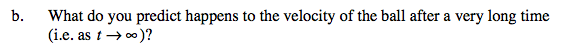Note: t = 100 seconds is a very long time for a ball to be in motion. (This must be an unearthly situation!) Is s(t) increasing, decreasing or neither as t→∞? Is s(t) changing rapidly or not so rapidly? Explain.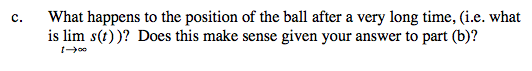Does a square root function (such as s(t)) have a horizontal asymptote as t→∞?